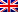# Hungarian algorithm

Contenus

## Hungarian algorithm

Also called Kühn's algorithm, the Hungarian algorithm, or Hungarian method solves assignment problems of the cost table type. Consider a number of machines and as many tasks. Each machine performs a task at a certain cost. The objective is to determine the machine on which each task will run, in parallel.

This problem being similar to a problem of coupling in a graph, it answers the criterion of König for the nodal coverage of minimum weight. The objective is therefore to find one item per row and column in the table such that the sum of the costs is minimal. If we start from a maximization problem, it will be necessary to reverse the costs in order to fall back on a minimization problem.

Let us take a simple example in order to show the process of the algorithm. Let 5 machines (row) and 5 tasks (column) give the following cost table:

 17 15 9 5 12 16 16 10 5 10 12 15 14 11 5 4 8 14 17 13 13 9 8 12 17

## Step 1 Hungarian algorithm: reduction of the initial table

We subtract from each line the smallest element of the line. The more we subtract from each column the smaller element of the column.

This standardization of machine and task costs makes it possible to have at least one zero per row and per column.

## Hungarian Algorithm Step 2: Find a Workable Solution

We are looking for the line with the fewest non-crossed zeros. In the event of a tie, we will take the highest line.

1. We surround one of the zeros of this line (arbitrarily the one furthest to the left).
2. All zeros in the same row and column as the one selected are crossed out.
3. We start again until all the zeros are boxed or crossed out.

If we have framed a zero by row and by column, we have an optimal solution. Otherwise we go to step 3.

## Step 3 Hungarian algorithm: creation of the pivots

The sub-table allows by subtraction to have a configuration making it possible to find an optimal solution. The construction of the pivots is done as follows:

1. We mark any line that does not contain any framed zero.
2. Any column with a crossed out zero is marked on a marked row.
3. We mark any row having a framed zero in a marked column.
4. Repeat 2 and 3 until no more changes.

A line is then drawn on any unmarked line and on any marked column. In our example, row 1 and 2 are marked, as well as column 4. Double-marked items are marked with a star.

## Step 4 Hungarian algorithm: modification of the table

The cells not crossed by a line constitute a partial table.

1. We subtract from all the boxes of this table the smallest element thereof.
2. This element is added to all the boxes of the table crossed out in both directions (accompanied by a star).

Steps 2 to 4 are repeated until an optimal result is obtained. In our example, we get an optimal result after the first iteration.

The minimum allocation is calculated from the initial table: 9 + 5 + 5 + 4 + 9 = 32.

The following figure shows the changes in the bipartite graph with the Hungarian algorithm.FRFRENRUES